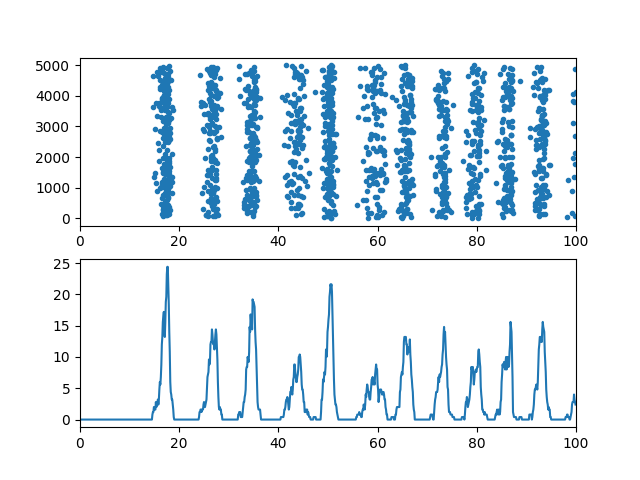# Example: Brunel_Hakim_1999¶

Note

You can launch an interactive, editable version of this example without installing any local files using the Binder service (although note that at some times this may be slow or fail to open):Dynamics of a network of sparsely connected inhibitory current-based integrate-and-fire neurons. Individual neurons fire irregularly at low rate but the network is in an oscillatory global activity regime where neurons are weakly synchronized.

Reference:
“Fast Global Oscillations in Networks of Integrate-and-Fire Neurons with Low Firing Rates” Nicolas Brunel & Vincent Hakim Neural Computation 11, 1621-1671 (1999)
from brian2 import *

N = 5000
Vr = 10*mV
theta = 20*mV
tau = 20*ms
delta = 2*ms
taurefr = 2*ms
duration = .1*second
C = 1000
sparseness = float(C)/N
J = .1*mV
muext = 25*mV
sigmaext = 1*mV

eqs = """
dV/dt = (-V+muext + sigmaext * sqrt(tau) * xi)/tau : volt
"""

group = NeuronGroup(N, eqs, threshold='V>theta',
reset='V=Vr', refractory=taurefr, method='euler')
group.V = Vr
conn = Synapses(group, group, on_pre='V += -J', delay=delta)
conn.connect(p=sparseness)
M = SpikeMonitor(group)
LFP = PopulationRateMonitor(group)

run(duration)

subplot(211)
plot(M.t/ms, M.i, '.')
xlim(0, duration/ms)

subplot(212)
plot(LFP.t/ms, LFP.smooth_rate(window='flat', width=0.5*ms)/Hz)
xlim(0, duration/ms)

show()